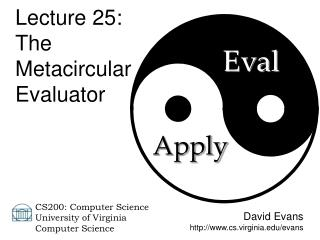DownloadDownload PresentationDavid Evans cs.virginia/evans

# David Evans cs.virginia/evans

Download Presentation## David Evans cs.virginia/evans

- - - - - - - - - - - - - - - - - - - - - - - - - - - E N D - - - - - - - - - - - - - - - - - - - - - - - - - - -
##### Presentation Transcript

1. Lecture 25: The Metacircular Evaluator Eval David Evans http://www.cs.virginia.edu/evans Apply CS200: Computer Science University of Virginia Computer Science

2. Menu • Metacircular Evaluator Core Review • Implementing Environments CS 200 Spring 2003

3. Environmental Model of Evaluation • To evaluate a combination, evaluate all the subexpressions and apply the value of the first subexpression to the values of the other subexpressions. • To apply a compound procedure to a set of arguments, evaluate the body of the procedure in a new environment. The parent of the new environment is the procedure’s environment; the frame is a new frame that contains places with the formal parameters bound to the arguments. CS 200 Spring 2003

4. meval (define (meval expr env) (cond ((self-evaluating? expr) expr) ((variable? expr) (environment-lookup-name expr env)) ((lambda? expr) (make-procedure (lambda-parameters expr) (lambda-body expr) env)) ((application? expr) (mapply (meval (application-operator expr) env) (map (lambda (subexpr) (meval subexpr env)) (application-operands expr)))) (else (error "Unknown expression: " exp)))) CS 200 Spring 2003

5. mapply (define (mapply procedure operands) (cond ((primitive-procedure? procedure) (apply-primitive procedure operands)) ((compound-procedure? procedure) (meval-sequence (procedure-body procedure) (extend-environment (procedure-parameters procedure) operands (procedure-environment procedure)))) (else (error “Can’t apply: " procedure)))) CS 200 Spring 2003

6. Environments CS 200 Spring 2003

7. From Lecture 19: global environment (define nest (lambda (x) (lambda (x) (+ x x)))) > ((nest 3) 4) + : #<primitive:+> nest: x : 3 parameters: xbody: (lambda (x) (+ x x)) 3 x : (nest 3) ((lambda (x) (+ x x)) 4) 4 x : CS 200 Spring 2003 (+ x x)

8. Representing Environments An environment is a frame and a parent. 4 x : (define (make-new-environment frame env) (cons frame env)) CS 200 Spring 2003

9. Environment Procedures (define (first-frame env) (car env)) (define (enclosing-environment env) (cdr env)) CS 200 Spring 2003

10. Representing Frames A frame is a list of name-value pairs. 3 y : 4 x : (define (make-empty-frame) (list)) CS 200 Spring 2003

11. Environmental Model of Evaluation • To evaluate a combination, evaluate all the subexpressions and apply the value of the first subexpression to the values of the other subexpressions. • To apply a compound procedure to a set of arguments, evaluate the body of the procedure in a new environment. The parent of the new environment is the procedure’s environment; the frame is a new frame that contains places with the formal parameters bound to the arguments. CS 200 Spring 2003

12. mapply (define (mapply procedure operands) (cond ((primitive-procedure? procedure) (apply-primitive procedure operands)) ((compound-procedure? procedure) (meval-sequence (procedure-body procedure) (extend-environment (procedure-parameters procedure) operands (procedure-environment procedure)))) (else (error “Can’t apply: " procedure)))) CS 200 Spring 2003

13. extend-environment (define (extend-environment names values env) (make-new-environment (map (lambda (name value) (cons name value)) names values) env)) CS 200 Spring 2003

14. meval (define (meval expr env) (cond ((self-evaluating? expr) expr) ((variable? expr) (environment-lookup-name expr env)) ((lambda? expr) (make-procedure (lambda-parameters expr) (lambda-body expr) env)) ((application? expr) (mapply (meval (application-operator expr) env) (map (lambda (subexpr) (meval subexpr env)) (application-operands expr)))) (else (error "Unknown expression: " exp)))) CS 200 Spring 2003

15. environment-lookup-name (define (environment-lookup-name name env) (if (null? env) (error "No binding " name) (if (frame-contains? name (first-frame env)) (frame-lookup-name name (first-frame env)) (environment-lookup-name name (enclosing-environment env))))) CS 200 Spring 2003

16. frame procedures (define (frame-contains? name frame) (insertlg (lambda (var result) (if (eq? (car var) name) #t result)) frame #f)) (define (frame-lookup-name name frame) (if (null? frame) (error "Name not found:" name) (if (eq? (car (car frame)) name) (cdr (car frame)) (frame-lookup-name name (cdr frame))))) CS 200 Spring 2003

17. meval (define (meval expr env) (cond ((self-evaluating? expr) expr) ((variable? expr) (environment-lookup-name expr env)) ((lambda? expr) (make-procedure (lambda-parameters expr) (lambda-body expr) env)) ((application? expr) (mapply (meval (application-operator expr) env) (map (lambda (subexpr) (meval subexpr env)) (application-operands expr)))) (else (error "Unknown expression: " exp)))) CS 200 Spring 2003

18. mapply (define (mapply procedure operands) (cond ((primitive-procedure? procedure) (apply-primitive procedure operands)) ((compound-procedure? procedure) (meval-sequence (procedure-body procedure) (extend-environment (procedure-parameters procedure) operands (procedure-environment procedure)))) (else (error “Can’t apply: " procedure)))) CS 200 Spring 2003

19. procedure procedures (define (make-procedure parameters body environment) (list 'procedure parameters body environment)) (define (compound-procedure? expr) (tagged-list? expr 'procedure)) (define (procedure-parameters procedure) (cadr procedure)) (define (procedure-body procedure) (caddr procedure)) (define (procedure-environment procedure) (cadddr procedure)) (define (tagged-list? expr tag) (if (pair? expr) (eq? (car expr) tag) #f)) CS 200 Spring 2003

20. meval (define (meval expr env) (cond ((self-evaluating? expr) expr) ((variable? expr) (environment-lookup-name expr env)) ((lambda? expr) (make-procedure (lambda-parameters expr) (lambda-body expr) env)) ((application? expr) (mapply (meval (application-operator expr) env) (map (lambda (subexpr) (meval subexpr env)) (application-operands expr)))) (else (error "Unknown expression: " exp)))) CS 200 Spring 2003

21. self-evaluating? (define (self-evaluating? expr) (or (number? expr) (string? expr) (primitive-procedure? expr))) CS 200 Spring 2003

22. Primitive Procedures (define (primitive-procedure? expr) (tagged-list? expr 'primitive-procedure)) (define (make-primitive-procedure expr) (list 'primitive-procedure expr)) (define (primitive-procedure-procedure procedure) (cadr procedure)) CS 200 Spring 2003

23. the-global-environment (define the-empty-environment '()) (define the-global-environment (make-new-environment (list (cons '+ (make-primitive-procedure +)) (cons '* (make-primitive-procedure *)) (cons '- (make-primitive-procedure -)) ) the-empty-environment)) CS 200 Spring 2003

24. Charge • This is powerful: once we have an metacircular evaluator, we can easily make changes to the language! • Friday: practice on problem classification problems CS 200 Spring 2003